This time we decided to provide you with the most simple Google Sheets functions that you definitely need to learn. They will not only help you with the plain calculations but also contribute to extending your knowledge of building Google Sheets formulas.

## How to build Google Sheets formulas

Whatever article Google Sheets formulas I've seen, they all start with an explanation of two main aspects: what is a function and what is a formula. Luckily, we've already covered this in a special starter guide on Google Sheets formulas. Besides, it sheds some light on cell references and various operators. If you haven't seen it yet, it's high time to check it out.

Another article of ours shares everything you need to know to be able to add your very first formulas in Google Sheets, reference other cells and sheets, or copy formulas down the column.

Once you've got these covered, you'll have no problems using variations of basic Google Sheets functions described below.

## 12 most useful Google Sheets functions

It's not a secret that there are tens of functions in spreadsheets, each with its own features and for its own purpose. But this doesn't mean you know nothing about electronic tables if you don't master them all.

There's a small set of Google Sheets functions that will let you last long enough without digging dip into spreadsheets. Allow me to introduce them to you.

Tip. If your task is super tricky and basic Google Sheets formulas are not what you're looking for, check out our collection of quick tools – Power Tools.

Now, this is one of those Google Sheets functions that you have to learn one way or the other. It adds up several numbers and/or cells and returns their total:

=SUM(value1, [value2, ...])
• value1 is the first value to sum. It can be a number, a cell with a number, or even a range of cells with numbers. This argument is required.
• value2, ... – all other numbers and/or cells with numbers you'd like to add to value1. The square brackets hint that this one is optional. And in this particular case, it can be repeated several times.

Tip. You can find the functions among standard instruments on Google Sheets toolbar: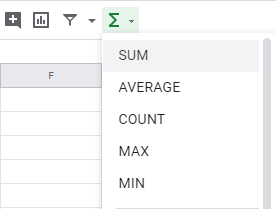I can create various Google Sheets SUM formulas like these:

`=SUM(2,6)` to calculate two numbers (the number of kiwis for me)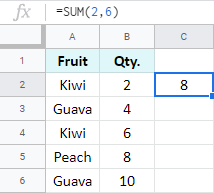`=SUM(2,4,6,8,10)` to calculate several numbers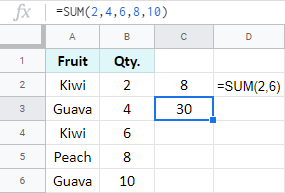`=SUM(B2:B6)` to add up multiple cells within the range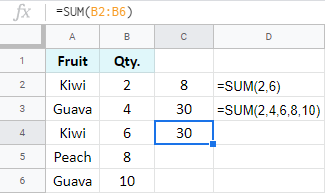Tip. There's a trick the function lets you do in order to swiftly add cells in Google Sheets in a column or a row. Try entering the SUM function right below the column you want to total or to the right of the row of interest. You'll see how it suggests the correct range instantly: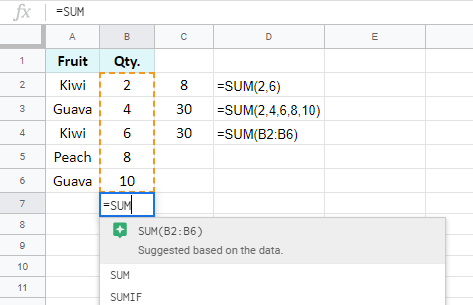### COUNT & COUNTA

This couple of Google Sheets functions will let you know how many cells of different contents your range contains. The only difference between them is that Google Sheets COUNT works only with numeric cells, while COUNTA counts cells with text as well.

So, to total all cells with numbers only, you use COUNT for Google Sheets:

=COUNT(value1, [value2, ...])
• value1 is the first value or range to check.
• value2 – other values or ranges to use for counting. As I told you before, square brackets mean that the function may get by without value2.

Here's the formula I've got:

`=COUNT(B2:B7)`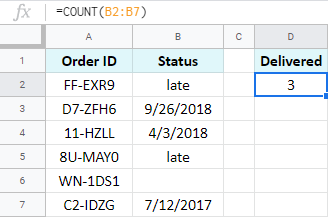If I am to get all orders with a known status, I will have to use another function: COUNTA for Google Sheets. It counts all non-empty cells: cells with text, numbers, dates, booleans – you name it.

=COUNTA(value1, [value2, ...])

The drill with its arguments is the same: value1 and value2 represent values or ranges to process, value2 and the following are optional.

Notice the difference:

`=COUNTA(B2:B7)`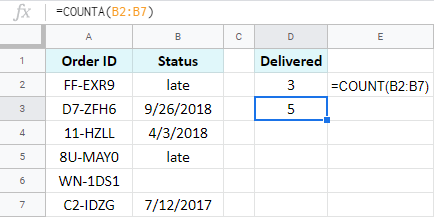COUNTA in Google Sheets takes all cells with contents into account, whether numbers or not.

### SUMIF & COUNTIF

Whilst SUM, COUNT, and COUNTA calculate all records you feed to them, SUMIF and COUNTIF in Google Sheets process those cells that meet specific requirements. The parts of the formula will be as follows:

=COUNTIF(range, criterion)
• range to count – required
• criterion to consider for counting – required
=SUMIF(range, criterion, [sum_range])
• range to scan for values related to the criterion – required
• criterion to apply to the range – required
• sum_range – the range to add up records from if it differs from the first range – optional

For example, I can find out the number of orders that fall behind schedule:

`=COUNTIF(B2:B7,"late")`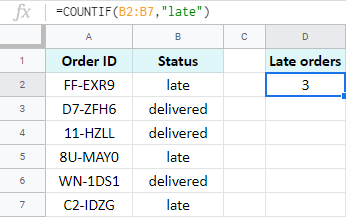Or I can get the total quantity of kiwis only:

`=SUMIF(A2:A6,"Kiwi",B2:B6)`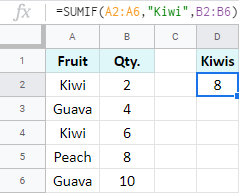In math, the average is the sum of all numbers divided by their count. Here in Google Sheets the AVERAGE function does the same: it evaluates the entire range and finds the average of all numbers ignoring the text.

=AVERAGE(value1, [value2, ...])

You can type in multiple values or/and ranges to consider.

If the item is available for purchase in different stores at different prices, you can tally the average price:

`=AVERAGE(B2:B6)`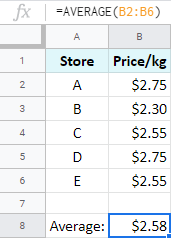### Google Sheets MAX & MIN functions

The names of these miniature functions speak for themselves.

Use Google Sheets MIN function to return the minimum number from the range:

`=MIN(B2:B6)`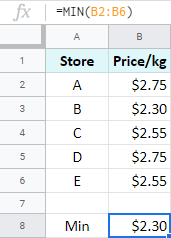Tip. To find the lowest number ignoring zeros, put the IF function inside:

`=MIN(IF(\$B\$2:\$B\$6<>0,\$B\$2:\$B\$6))`

Use Google Sheets MAX function to return the maximum number from the range:

`=MAX(B2:B6)`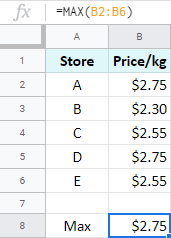Tip. Want to ignore zeros here as well? Not a problem. Just add another IF:

`=MAX(IF(\$B\$2:\$B\$6<>0,\$B\$2:\$B\$6))`

Easy peasy lemon squeezy. :)

Though IF function in Google Sheets is quite popular and commonly used, for some reasons it keeps confusing and puzzling its users. Its main purpose is to help you work out conditions and return different results accordingly. It is also often referred to as Google Sheets "IF/THEN" formula.

=IF(logical_expression, value_if_true, value_if_false)
• logical_expression is the condition itself that has two possible logical outcomes: TRUE or FALSE.
• value_if_true is whatever you want to return if your condition is met (TRUE).
• otherwise, when it's not met (FALSE), value_if_false is returned.

Here's a plain example: I'm evaluating ratings from feedback. If the number received is less than 5, I want to label it as poor. But if the rating is greater than 5, I need to see good. If I translate this to the spreadsheet language, I'll get the formula I need:

`=IF(A6<5,"poor","good")`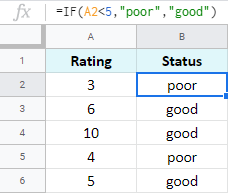### AND, OR

These two functions are purely logical.

Google spreadsheet AND function checks if all its values are logically correct, while Google Sheets OR function – if any of the provided conditions are true. Otherwise, both will return FALSE.

To be honest, I don't remember using these much on their own. But both are used in other functions and formulas, especially with the IF function for Google Sheets.

Adding Google Sheets AND function to my condition, I can check ratings in two columns. If both numbers are greater than or equal to 5, I mark the total request as "good", or else "poor":

`=IF(AND(A2>=5,B2>=5),"good","poor")`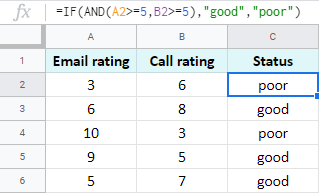But I can also change the condition and mark the status good if at least one number of two is more than or equal to 5. Google Sheets OR function will help:

`=IF(OR(A2>=5,B2>=5),"good","poor")`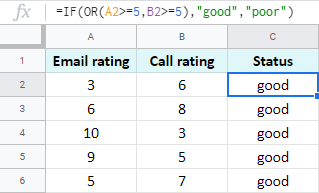If you need to merge records from several cells into one without losing any of the data, you should use Google Sheets CONCATENATE function:

=CONCATENATE(string1, [string2, ...])

Whatever characters, words, or references to other cells you give to the formula, it will return everything in one cell:

`=CONCATENATE(A2,B2)`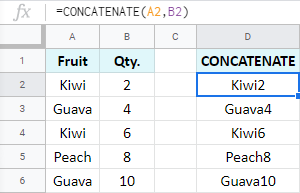The function also lets you separate combined records with chars of your choice, like this:

`=CONCATENATE(A2,", ",B2)`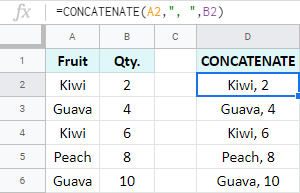You can quickly check the range for any extra spaces using the TRIM function:

=TRIM(text)

Enter the text itself or a reference to a cell with text. The function will look into it and not only trim all leading and trailing spaces but will also reduce their number between words to one: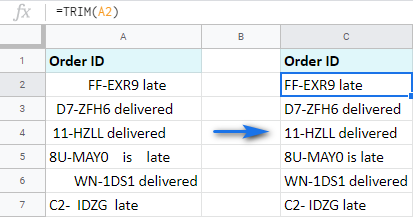### TODAY & NOW

With their help, you will insert today's date and time formulas in Google Sheets and they will update themselves whenever you access the document. I truly cannot imagine the simplest function than these two:

• `=TODAY()` will show you the today's date.
• `=NOW()` will return both the today's date and the current time.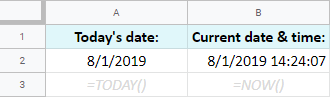If you're going to work with dates in electronic tables, Google Sheets DATE function is a must-learn.

When building different formulas, sooner or later you will notice that not all of them recognize dates entered as they are: 12/8/2019.

Besides, the locale of the spreadsheet dictates the format of the date. So the format you're used to (like 12/8/2019 in the US) may not be recognized by other users' Sheets (e.g. with the locale for the UK where dates look like 8/12/2019).

To avoid that, it's highly recommended to use the DATE function. It converts whatever day, month, and year you enter into a format that Google will always understand:

=DATE(year, month, day)

For example, if I were to subtract 7 days from my friend's birthday to know when to start preparing, I'd use the formula like this:

`=DATE(2019,9,17)-7`

Or I could make the DATE function return the 5th day of the current month and year:

`=DATE(YEAR(TODAY()),MONTH(TODAY()),5)`

And finally, the VLOOKUP function. That same function that keeps lots of Google Sheets users in terror. :) But the truth is, you only need to break it down once – and you won't remember how you lived without it.

Google Sheets VLOOKUP scans one column of your table in search of a record you specify and pulls the corresponding value from another column from that same row:

=VLOOKUP(search_key, range, index, [is_sorted])
• search_key is the value to look for
• range is the table where you need to search
• index is the number of the column where related records will be pulled from
• is_sorted is optional and used to hint that the column to scan is sorted

I have a table with fruits and I want to know how much oranges cost. For that, I create a formula that will look for Orange in the first column of my table and return the corresponding pricing from the third column:

`=VLOOKUP("Orange",A1:C6,3)`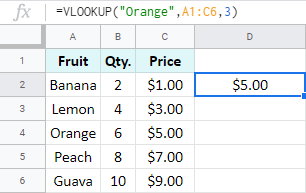## Modify multiple Google Sheets formulas quickly with a special tool

We also have a tool that helps you modify multiple Google Sheets formulas within the selected range at once. It's called Formulas. Let me show you how it works.

I have a small table where I used SUMIF functions to find the total of each fruit: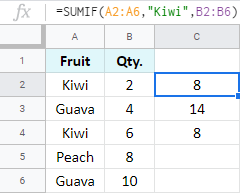I want to multiply all totals by 3 to restock. So I select the column with my formulas and open the add-on.

Note. Since the utility is part of Power Tools, you need to install it first. You will find the tool right at the bottom of the pane: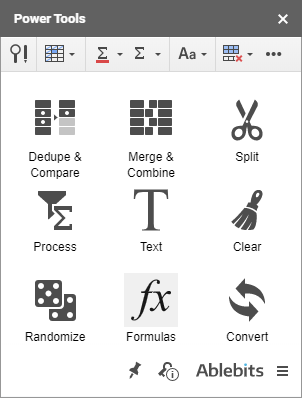Then I choose the option to Modify all selected formulas, add *3 at the end of the formula sample, and click Run. You can see how the totals change accordingly – all in one go: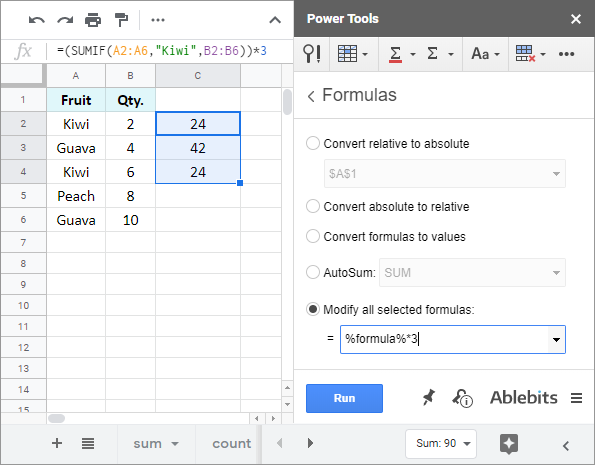## You may also be interested in

1. Veru useful, thanks!

2. Excellent breakdown. Very useful.

• Thank you for your feedback!

3. Thanks a lot to share such a great information